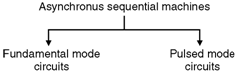Home > finite state machines > Asyncronous FSM Design > Modes of Asynchronous Sequential Machines

# Modes of Asynchronous Sequential Machines

Depending on the type of input variables, the way they are allowed to change etc. the asynchronous sequential circuits are classified into two categories shown below,Fig1-Modes-of-Asynchronous-Sequential-Machines

Asynchronous machine model :

Figure below shows the model of asynchronous sequential machine. It covers both fundamental and pulsed mode circuits. In Figure, â€œIâ€ represents the input variables, the excitation inputs to the memory (latches) is denoted by â€œEâ€, the memory output is labelled by â€œSâ€ and the output variables are denoted by â€œOâ€. Note that latches are used as memory elements and not the flip flops.Fig1-Modes-of-Asynchronous-Sequential-Machines

### Fundamental Mode Asynchronous Circuits :

The fundamental mode asynchronous circuit design is based on the following assumptions :

1. The inputs (I) to the synchronous circuits change only when the circuit is stable, that means when the state variables (S) are not in their transition state.
2. Another assumption is that the inputs are levels and not pulses.
3. The state variables in these circuits are characterized as delay elements. Delay may be introduced by a latch or simply the propagation delay inherent in the logic gates used for realizing the asynchronous circuits.

Total state :

• The behaviour of an asynchronous machine is determined by defining the total state.
• The total state is divided into two parts.
1. The input state (I).
2. The internal or secondary state (value of S).
• Thus the total state is defined by the value of (I, S). The total state can be either stable or unstable.

Stable total state :

• A stable total state is defined as the state which produces no additional state transitions. If I does not change then (I, S) remains stable.
• A total state transition occurs when a given input state (I) causes the excitation of memory to produce a secondary state (S).
• The secondary state outputs (S) are applied to the output logic (p) as well as to the input (f) to produce the memory excitation inputs (E).
• When the inputs to the combinational excitation logic do not produce any new values for the secondary states (S) the machine is said to be stable.
• The time delay required to reach the stable state depends on the following factors :
1. Excitation.
2. Memory logic delays and
3. State assignment.
• A large number of secondary state changes could occur before the machine reaches a stable total state.
• Note that in the fundamental mode model, the input state (I) is not allowed to change until the circuit has reached a stable total state.
• This is because if changes in (I) were allowed before a total stable state were reached, then the circuit may never reach a stable condition at all.
• One more important requirement of the fundamental mode asynchronous machine is that only one input variable can change at a given time. If this requirement is not satisfied, then the asynchronous circuit can make erroneous total state transitions.

Pulse Mode Asynchronous Circuits :

• In the pulsed mode, the input variables are allowed to be applied in the form of pulses, rather than in the form of levels.
• But the width of input pulses is a critical parameter. There are two restrictions on the width of the input pulses.
1. The first requirement is that the pulses should be long enough so that the circuit can respond to them.
2. The second requirement is that the pulses should not be too long so that they are still present after the new secondary state is reached.
• The second requirement is that the pulses should not be too long so that they are still present after the new secondary state is reached.
• The base of calculating the minimum pulse width is the propagation delay of the excitation logic.
• The maximum pulse width is calculated based on the total propagation delay through the excitation logic and the memory.